Question:

How can I perform set operations like union, intersect etc. on data sets with time interval information e.g. alarm start and end?

Solution:

In the article "Duration calculations of overlapping periods" we have presented how you can calculate the total duration of multiple periods.

If you are not interested in the total duration, but you want to perform set operations on these periods without loosing detailed information of each period you can use the functions we have prepared for you.

Below you will find functions for the following operations:

• periods2events
• intersecting_periods
• unified_periods
• nonoverlapping_periods_simple
• diff_periods
• nonoverlapping_periods

Short description of each function:

periods2events:

This function converts the input data frame where each observation consists of category, start and stop of the time interval in a data frame in form of an event list where each observation consists of the category, the timestamp of the event, the information if a rising or falling edge occurs and the information how many parallel intervals are at this moment.intersecting_periods:

This function returns all time intervals where intervals of all categories are overlapping.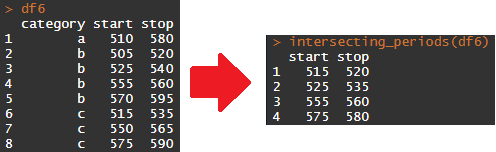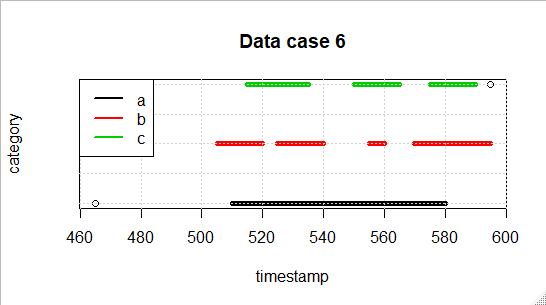unified_periods:

This function returns the unified periods of all intervalls and categories.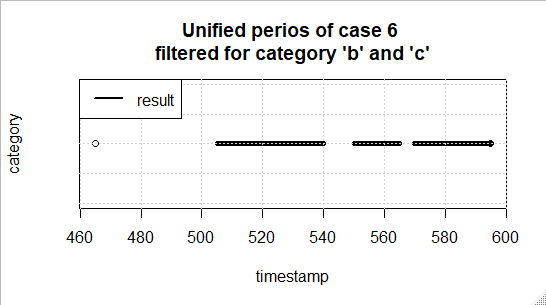nonoverlapping_periods_simple:

This function returns the non-overlapping periods considering 2 priorities. You can specify the high priority category with the "prio" parameter. If this parameter is not set, the first existing category in the input data in alphanumerical order will be taken as high priority. If there are overlapping periods of the high and low priority category the overlapping time is only accounted as the high priority category and cut from the low priority category. If there are more than 2 categories all low priority categories will be unified first and labelled with the alphanumerically ordered first low priority category.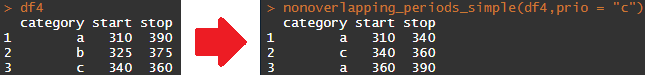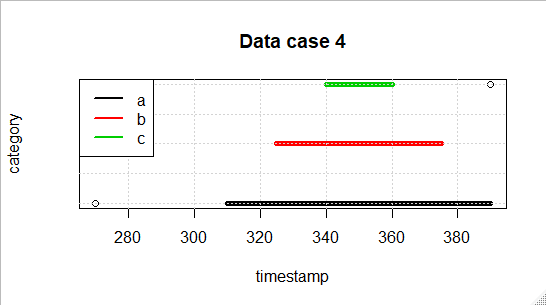diff_periods:

This function returns the remaining intervals of the category defined by "minuend" with all overlaps with other categories removed. If there is no minend defined the alphanumerically first category included in the input data will be taken as minuend.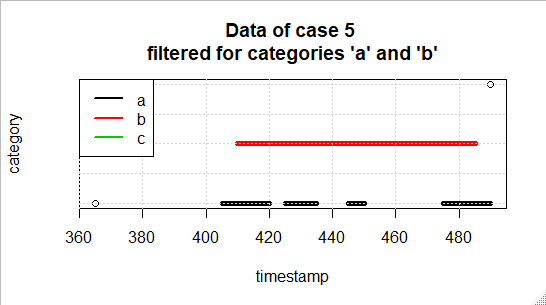nonoverlapping_periods:

This function returns the non-overlapping periods considering all priorities. You can specify the priority of each category with the "priorities" parameter. Where the first entry in this vector has the highest priority and the last entry has the lowest priority. If this parameter is not set, the alphanumerical order will be taken as the priority ranking. If not all categories within the input data set are mentioned in the priority ranking, the missing categories are ignored. Like in the function "nonoverlapping_periods_simple" the overlapping periods of different categories are only accounted as a period of the category with the higher priority and cut from the low priority category.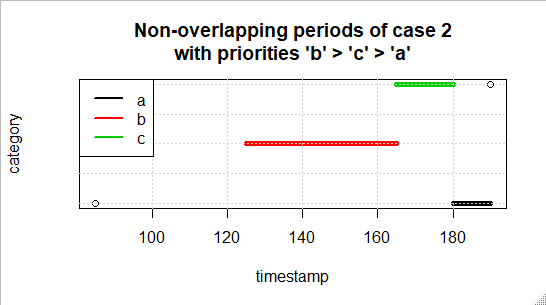Function definitions in R:

NOTE: To run the functions given below you have to load the "dplyr" and "tidyr" packages!

# ----------------------------------------
# ----- Period Calculation Functions -----
# ----------------------------------------

# -----------------------------------
# Function name:    periods2events
#
# Inputs:           data ... data frame with the three variables/columns category, start and stop
#
# Outputs:          data frame with four variables/columns category, timestamp, edge, parallels
#
# Description:      This function converts the input data frame where each observation consists of category, start and stop of the time interval
#                   in a data frame in form of an event list where each observation consists of the category, the timestamp of the event,
#                   the information if a rising or falling edge occurs and the information how many parallel intervals are at this moment.
#
# Required custom
# functions:        none
# -----------------------------------
periods2events <- function (data)
{
log <- data.frame(category=data\$category, timestamp=c(data\$start, data\$stop), edge=rep(c(1, -1), each=nrow(data)))
log_ordered <- log[order(log\$timestamp),] %>%
mutate(parallels = cumsum(edge))
log_ordered <- log_ordered[!duplicated(log_ordered['category','timestamp'], fromLast=T),]
return(log_ordered)
}

# -----------------------------------
# Function name:    intersecting_periods
#
# Inputs:           data ... data frame with the three variables/columns category, start and stop
#                   optional: filter ... vector with categories for which the data should be filtered
#
# Outputs:          data frame with two variables/colums start and stop
#
# Description:      This function returns all time intervals where intervals of all categories are overlapping.
#
# Required custom
# functions:        periods2events
# -----------------------------------
intersecting_periods <- function (data, filter = unique(data\$category))
{
filter <- as.character(filter)

log_ordered <- periods2events(data %>% filter(category %in% filter))

if(any(table(log_ordered\$category) == nrow(log_ordered)))
{
log_intersect <- data.frame(start=NULL, stop=NULL)
}
else
{
pos_intersect <- which(log_ordered\$parallels == length(unique(log_ordered\$category)))
log_intersect <- data.frame(start=log_ordered\$timestamp[pos_intersect], stop=log_ordered\$timestamp[pos_intersect+1]) %>%
filter(start!=stop)
}
return(log_intersect)
}

# -----------------------------------
# Function name:    unified_periods
#
# Inputs:           data ... data frame with the three variables/columns category, start and stop
#                   optional: filter ... vector with categories for which the data should be filtered
#
# Outputs:          data frame with two variables/colums start and stop
#
# Description:      This function returns the unified periods of all intervalls and categories.
#
# Required custom
# functions:        periods2events
# -----------------------------------
unified_periods <- function (data, filter = unique(data\$category))
{
filter <- as.character(filter)

data_filtered <- data %>% filter(category %in% filter)
if(nrow(data_filtered) == 0)
{
log_unified <- data.frame(start=NULL, stop=NULL)
}
else
{
log_ordered <- periods2events(data_filtered)
pos_zero <- which(log_ordered\$parallels == 0)
log_unified <- data.frame( start = c(log_ordered\$timestamp, log_ordered\$timestamp[head(pos_zero, -1)+1]),
stop = log_ordered\$timestamp[pos_zero])
}
return(log_unified)
}

# -----------------------------------
# Function name:    nonoverlapping_periods_simple
#
# Inputs:           data ... data frame with the three variables/columns category, start and stop
#                   optional: filter ... vector with categories for which the data should be filtered
#                   optional: prio ... category with the higher priority
#
# Outputs:          data frame with three variables/colums category, start and stop
#
# Description:      This function returns the non-overlapping periods considering 2 priorities.
#                   You can specify the high priority category with the "prio" parameter. If this parameter is not set, the first existing
#                   category in the input data in alphanumerical order will be taken as high priority.
#                   If there are overlapping periods of the high and low priority category the overlapping time is only accounted as the high
#                   priority category and cut from the low priority category.
#                   If there are more than 2 categories all low priority categories will be unified first and labelled with the alphanumerically
#                   ordered first low priority category.
#
# Required custom
# functions:        periods2events, unified_periods
# -----------------------------------
nonoverlapping_periods_simple <- function (data, filter = unique(data\$category), prio = filter[order(filter)])
{
prio <-  as.character(prio)
filter <- as.character(filter)

low_prio <- filter[order(filter)]
low_prio <- low_prio[low_prio != prio]

data_filtered <- data %>% filter(category %in% filter)

log_high_prio <- data_filtered %>% filter(category == prio)

log_low_prio <- data_filtered %>%
filter(category %in% low_prio) %>%
unified_periods() %>%
mutate(category = low_prio)

log_ordered <- bind_rows(log_high_prio, log_low_prio) %>%
periods2events()

log_ordered_periods <- log_ordered %>%
mutate( a_on = cumsum(ifelse(category == prio,edge,0)),
b_on = cumsum(ifelse(category == low_prio,edge,0)))
log_non_overlap <- log_ordered_periods %>%
filter(category == prio | (category == low_prio & a_on == 0))
log_non_overlap <- bind_rows(log_non_overlap, log_non_overlap %>%
filter(category == prio & b_on == 1) %>%
mutate(category = low_prio, edge = -edge))
log_non_overlap <- log_non_overlap %>%
select(category, timestamp, edge) %>%
arrange(timestamp)

start = log_non_overlap\$timestamp[log_non_overlap\$edge==1],
stop = log_non_overlap\$timestamp[log_non_overlap\$edge==-1])
}

# -----------------------------------
# Function name:    diff_periods
#
# Inputs:           data ... data frame with the three variables/columns category, start and stop
#                   optional: filter ... vector with categories for which the data should be filtered
#                   optional: minuend ... category from which the periods of the other categories should be subtracted
#
# Outputs:          data frame with three variables/colums category, start and stop
#
# Description:      This function returns the remaining intervals of the category defined by "minuend" with all overlaps with other categories removed.
#                   If there is no minend defined the alphanumerically first category included in the input data will be taken as minuend.
#
# Required custom
# functions:        periods2events, unified_periods, nonoverlapping_periods_simple
# -----------------------------------
diff_periods <- function (data, filter = unique(data\$category), minuend = filter[order(filter)])
{
minuend <-  as.character(minuend)
filter <- as.character(filter)

data_filtered <- data %>% filter(category %in% filter)
data_filtered\$category <- as.character(data_filtered\$category)

if(is.na(table(data\$category)[minuend]))
{
return(data.frame(start=NULL, stop=NULL))
}

if(table(data\$category)[minuend] == nrow(data))
{
return(data)
}

log_subtrahend <- data_filtered %>%
filter(category != minuend) %>%
unified_periods() %>%
mutate(category = filter[filter != minuend])

log_minuend <- data_filtered %>%
filter(category == minuend)

log_data <- bind_rows(log_subtrahend, log_minuend) %>%
arrange(start)

return(nonoverlapping_periods_simple(log_data,
filter = c(minuend, filter[filter != minuend]),
prio = filter[filter != minuend]) %>% filter(category == minuend))
}

# -----------------------------------
# Function name:    nonoverlapping_periods
#
# Inputs:           data ... data frame with the three variables/columns category, start and stop
#                   optional: filter ... vector with categories for which the data should be filtered
#                   optional: priorities ... vector of categories where the position within the category defines the priority starting with highest priority
#
# Outputs:          data frame with three variables/colums category, start and stop
#
# Description:      This function returns the non-overlapping periods considering all priorities.
#                   You can specify the priority of each category with the "priorities" parameter. Where the first entry in this vector has the highest
#                   priority and the last entry has the lowest priority. If this parameter is not set, the alphanumerical order will be taken as the
#                   priority ranking.
#                   If not all categories within the input data set are mentioned in the priority ranking, the missing categories are ignored.
#                   Like in the function "nonoverlapping_periods_simple" the overlapping periods of different categories are only accounted as a period
#                   of the category with the higher priority and cut from the low priority category.
#
# Required custom
# functions:        periods2events, unified_periods, diff_periods, nonoverlapping_periods_simple
# -----------------------------------
nonoverlapping_periods <- function (data, filter = unique(data\$category), priorities = filter[order(filter)])
{
priorities <-  as.character(priorities)
filter <- as.character(filter)

data_filtered <- data %>% filter(category %in% filter)
data_filtered\$category <- as.character(data_filtered\$category)

log_non_overlap <- NULL

for(i in length(priorities):1)
{
if(i > 1)
{
log_higher_prio <- data_filtered %>%
filter(category %in% priorities[1:(i-1)]) %>%
unified_periods(filter = filter) %>%
mutate(category = "high")

log_lower_prio <- data_filtered %>%
filter(category == priorities[i])

log_bind_prio <-  bind_rows(log_higher_prio, log_lower_prio) %>%
arrange(start)

minu <- priorities[i]

log_non_overlap_prio <- diff_periods(log_bind_prio, minuend = minu)
}
else if(i != 0)
{
log_non_overlap_prio <- data_filtered %>% filter(category == priorities)
}

if(is.null(log_non_overlap))
{
log_non_overlap <- log_non_overlap_prio
}
else
{
log_non_overlap <- bind_rows(log_non_overlap,log_non_overlap_prio)
}
}

return(log_non_overlap %>% arrange(start))
}

In the attachments of this article you can also find a R-Script which demonstrates the functionality of these functions.

If you encounter any problems with these function or if you have any problems applying them, don't hesitate to contact our customer support team.